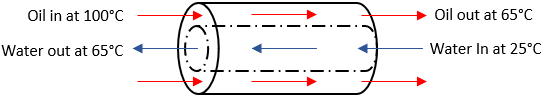## Heat Exchanger

In the diagram below, water flows into an inner pipe at 25°C. An outer pipe encapsulates the inner water pipe with oil entering at 100°C and 3 kg/s. If water exits the heat exchanger at 65°C, what is the mass flow rate (kg/s) of the water? Note the specific heat (kJ/kg·K) for both water and oil are 4.186 and 3.5 respectively.Hint
In a heat exchanger, the rate of heat transfer associated with either steam, an incompressible fluid, or ideal gas with constant specific heat flows is
$$\dot{Q}=\dot{m}c_p(\Delta T)$$$where $$c_p$$ is the specific heat (at constant pressure), $$\dot m$$ is the mass flow rate, and $$\Delta T$$ is the change in temperature. Hint 2 Because the heat transfer between the water & oil occurs within the same system, $$\dot{Q}_{oil}=\dot{Q}_{water}$$ . In a heat exchanger, the rate of heat transfer associated with either steam, an incompressible fluid, or ideal gas with constant specific heat flows is $$\dot{Q}=\dot{m}c_p(\Delta T)$$$
where $$c_p$$ is the specific heat (at constant pressure), $$\dot m$$ is the mass flow rate, and $$\Delta T$$ is the change in temperature. Per the problem statement, we can write a rate of heat transfer equation for both the oil and water:
$$\dot{Q}_{water}=\dot{m}_{water}\times c_{p,water}(\Delta T_{water})$$$$$\dot{Q}_{oil}=\dot{m}_{oil}\times c_{p,oil}(\Delta T_{oil})$$$
Because the heat transfer between the water & oil occurs within the same system, $$\dot{Q}_{oil}=\dot{Q}_{water}$$ :
$$\dot{m}_{water}\times c_{p,water}(\Delta T_{water})=\dot{m}_{oil}\times c_{p,oil}(\Delta T_{oil})$$$$$\dot{m}_{water}=\dot{m}_{oil}\times \frac{c_{p,oil}}{c_{p,water}}\times \frac{(\Delta T_{oil})}{(\Delta T_{water})}$$$
$$\dot{m}_{water}=3\frac{kg}{s}\times \frac{3.5kJ/kg\cdot K}{4.186kJ/kg\cdot K}\times \frac{[(100+273K)-(65+273K)]}{[(65+273K)-(25+273K)]}$$$$$=3\frac{kg}{s}\times 0.836\times \frac{35}{40}=2.19\:kg/s$$$
2.19 kg/s